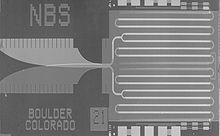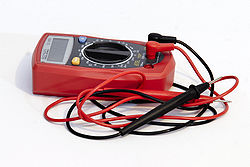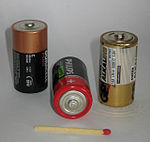# Volt

VoltJosephson junction array chip developed by NIST as a standard volt

The volt (symbol: V) is the SI derived unit for electric potential, electric potential difference, and electromotive force. The volt is named in honor of the Italian physicist Alessandro Volta (1745–1827), who invented the voltaic pile, possibly the first chemical battery.

## Definition

A single volt is defined as the difference in electric potential across a wire when an electric current of one ampere dissipates one watt of power. It is also equal to the potential difference between two points 1 meter apart in an electric field of 1 newton per coulomb. Additionally, it is the potential difference between two points that will impart one joule of energy per coulomb of charge that passes through it. It can be expressed in terms of SI units as follows:$\mbox{V} = \mbox{A} \cdot \Omega= \dfrac{\mbox{W}}{\mbox{A}} = \sqrt{\mbox{W} \cdot \Omega} = \dfrac{\mbox{J}}{\mbox{A} \cdot \mbox{s}} = \dfrac{\mbox{N} \cdot \mbox{m} }{\mbox{A} \cdot \mbox{s}} = \dfrac{\mbox{kg} \cdot \mbox{m}^2}{\mbox{C} \cdot \mbox{s}^2} = \dfrac{\mbox{N} \cdot \mbox{m}} {\mbox{C}} = \dfrac{\mbox{J}}{\mbox{C}}$

It can also be written using only the SI base units m, kg, s, and A as:$\mbox{V} = \dfrac{\mbox{kg} \cdot \mbox{m}^2}{\mbox{A} \cdot \mbox{s}^{3}}$

### Josephson junction definition

Between 1990 and 1997, the volt was calibrated using the Josephson effect for exact voltage-to-frequency conversion, combined with cesium-133 time reference, as decided by the 18th General Conference on Weights and Measures. The following value for the Josephson constant is used:

K{J-90} = 2e/h = 0.4835979 GHz/µV.

This is typically used with an array of several thousand or tens of thousands of junctions, excited by microwave signals between 10 and 80 GHz (depending on the array design). Empirically, several experiments have shown that the method is independent of device design, material, measurement setup, etc., and no correction terms are required in a practical implementation. However, as of July 2007, this is not the official BIPM definition of Volt.

## Water flow analogy

In the water flow analogy sometimes used to explain electric circuits by comparing them to water-filled pipes, voltage difference is likened to water pressure difference–the difference determines how quickly the electrons will travel through the circuit. Current (in amperes), in the same analogy, is a measure of the volume of water that flows past a given point per unit time (volumetric flow rate). The flow rate is determined by the width of the pipe (analogous to electrical conductivity), and the pressure difference between the front end of the pipe and the exit (analogous to voltage). The analogy extends to power dissipation: the power given up by the water flow is equal to flow rate times pressure, just as the power dissipated in a resistor is equal to current times the voltage drop across the resistor (watts = amperes × volts).

The relationship between voltage and current (in ohmic devices) is defined by Ohm's Law.

## Common voltagesA multimeter can be used to measure the voltage between two positions.1.5 V C-cell batteries

Nominal voltages of familiar sources:

Note: Where RMS (root mean square) is stated above, the peak voltage is$\sqrt{2}$ times greater than the RMS voltage for a sinusoidal signal centered around zero voltage.

## History of the volt

In 1800, as the result of a professional disagreement over the galvanic response advocated by Luigi Galvani, Alessandro Volta developed the so-called Voltaic pile, a forerunner of the battery, which produced a steady electric current. Volta had determined that the most effective pair of dissimilar metals to produce electricity is zinc and silver. In the 1880s, the International Electrical Congress, now the International Electrotechnical Commission (IEC), approved the volt as the unit for electromotive force. At that time, the volt was defined as the potential difference [i.e., what is nowadays called the "voltage (difference)"] across a conductor when a current of one ampere dissipates one watt of power.

The international volt was defined in 1893 as 1/1.434 of the emf of a Clark cell. This definition was abandoned in 1908 in favor of a definition based on the international ohm and international ampere until the entire set of "reproducible units" was abandoned in 1948.

Prior to the development of the Josephson junction voltage standard, the volt was maintained in national laboratories using specially constructed batteries called standard cells. The United States used a design called the Weston cell from 1905 to 1972.

This SI unit is named after Alessandro Volta. As with every SI unit whose name is derived from the proper name of a person, the first letter of its symbol is upper case (V). When an SI unit is spelled out in English, it should always begin with a lower case letter (volt), except where any word would be capitalized, such as at the beginning of a sentence or in capitalized material such as a title. Note that "degree Celsius" conforms to this rule because the "d" is lowercase. —Based on The International System of Units, section 5.2.

 Ampere Electric potential difference Rail traction voltage Ohm SI electromagnetism units SI prefix for unit prefixes

Wikimedia Foundation. 2010.

### Look at other dictionaries:

• volt — volt …   Dictionnaire des rimes

• volt — [ vɔlt ] n. m. • 1874; du nom du physicien Volta ♦ Métrol. Unité de mesure de potentiel, de différence de potentiel (ou tension) et de force électromotrice (symb. V). Le volt est la différence de potentiel existant entre deux points lorsque le… …   Encyclopédie Universelle

• volt — VOLT, volţi, s.m. (fiz.) Unitate de măsură a tensiunii electrice, a tensiunii electromotoare şi a diferenţei de potenţial, egală cu tensiunea de la capetele unui conductor care, străbătut de un curent de un amper, dezvoltă o putere de un watt. –… …   Dicționar Român

• Volt — Sn std. (19. Jh.) Onomastische Bildung. Neubildung zu Volta, dem Namen eines italienischen Physikers des 18./19. Jhs.    Ebenso nndl. volt, ne. volt, nfrz. volt, nschw. volt, nisl. volt. Name …   Etymologisches Wörterbuch der deutschen sprache

• Volt — Saltar a navegación, búsqueda Volt puede estar haciendo referencia a: Voltio, unidad del Sistema Internacional de voltaje y potencial eléctrico. Volt, modelo de utilitario híbrido de la marca Chevrolet. Obtenido de Volt Categoría:… …   Wikipedia Español

• volt — volt1 [vōlt] n. [Fr volte < It volta, a turn < VL * volvita, for L voluta, fem. pp. of volvere, to roll, turn about: see WALK] 1. a turning movement or gait of a horse, in which it moves sideways around a center 2. Fencing a leap to avoid a …   English World dictionary

• Volt — Volt, n. [F. volte; cf. It. volta. See {Vault}.] [1913 Webster] 1. (Man.) A circular tread; a gait by which a horse going sideways round a center makes two concentric tracks. [1913 Webster] 2. (Fencing) A sudden movement to avoid a thrust. [1913… …   The Collaborative International Dictionary of English

• Volt — Volt, n. [After Alessandro Volta, the Italian electrician.] (Elec.) The unit of electro motive force; defined by the International Electrical Congress in 1893 and by United States Statute as, that electro motive force which steadily applied to a… …   The Collaborative International Dictionary of English

• volt — sb. (fk.), volt (fork.: V) …   Dansk ordbog

• Volt — Volt, s. Elektrische Maßeinheiten, S. 641 …   Meyers Großes Konversations-Lexikon

• Volt — Volt, s. Maßsystem, absolutes, Bd. 6, S. 329 …   Lexikon der gesamten Technik